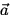# Gravitational Potential Energy

Definition: Gravitational Potential Energy ( PEg ) is given by:
 PEg = mgy, (3)
where m is the mass of an object, g is the acceleration due to gravity, and y is the distance the object is above some reference level.

The term energy'' is motivated by the fact that potential energy and kinetic energy are different aspects of the same thing (mechanical energy).

For Example: When an object is dropped from rest at some height above the earth's surface, it starts with some PEg but no KE. As the object falls towards the Earth, it loses PEg and gains KE. Just before the object hits the ground, it has lost all of its initial PEg but gained an equal amount of KE.

Proof: Find the work done by the force of gravity when an object falls from rest at position yi to yf = 0 . We have W = Fs , F = |m| = mg and s = (yi - yf) = yi . This gives, W = mgyi .

Combining with Eq.(5.2) gives 1/2m(vf2 - 0) = mgyi or PEi = KEf .Next: Potential Energy Stored in Up: Work and Energy Previous: Kinetic Energy and the